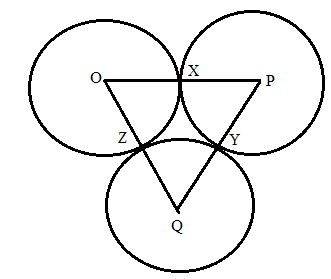Chapter 6.3, Problem 42EElementary Geometry For College St...

7th Edition
Alexander + 2 others
ISBN: 9781337614085

Solutions

Chapter
SectionElementary Geometry For College St...

7th Edition
Alexander + 2 others
ISBN: 9781337614085
Textbook Problem

Circles O, P, and Q are tangent (as shown for Exercise 40).If O X = 2 , P Y = 3 , Q Z = 1 , name:a) .The smallest angle of Δ P Q O b) .The smallest angle of Δ P Q OTo determine

a)

To find:

To name the smallest angle of ΔPQO if:

OX=2,PY=3,QZ=1

Explanation

Given that, circles O, P, and Q are tangent (as shown) at points X, Y, and Z..

Then the value of OX=2,PY=3,QZ=1.

The diagrammatic representation is given below,

To find the measures of the sides of the triangle ΔPQO is given below,

OP=OX+PXPQ=PY+QYOQ=QZ+OZ

We know that OX=OZ,PX=PY,QY=QZ since radius of the circle is same for all points on the circle.

Substitute the value of OX=2,PY=3,QZ=1 and OX=OZ,PX=PY,QY=Q

To determine

b)

To find:

To name the largest angle of ΔPQO if:

OX=2,PY=3,QZ=1

Still sussing out bartleby?

Check out a sample textbook solution.

See a sample solution

The Solution to Your Study Problems

Bartleby provides explanations to thousands of textbook problems written by our experts, many with advanced degrees!

Get Started

Sketch the graph of y=x24.

Calculus: An Applied Approach (MindTap Course List)

Expand each expression in Exercises 122. (4y2)y

Finite Mathematics and Applied Calculus (MindTap Course List)

In Problems 13-26, graph the solution of each system of inequalities. 19.

Mathematical Applications for the Management, Life, and Social Sciences

Find the matrix A if A=

Finite Mathematics for the Managerial, Life, and Social Sciences

True or False: If converges and converges, then converges.

Study Guide for Stewart's Single Variable Calculus: Early Transcendentals, 8th